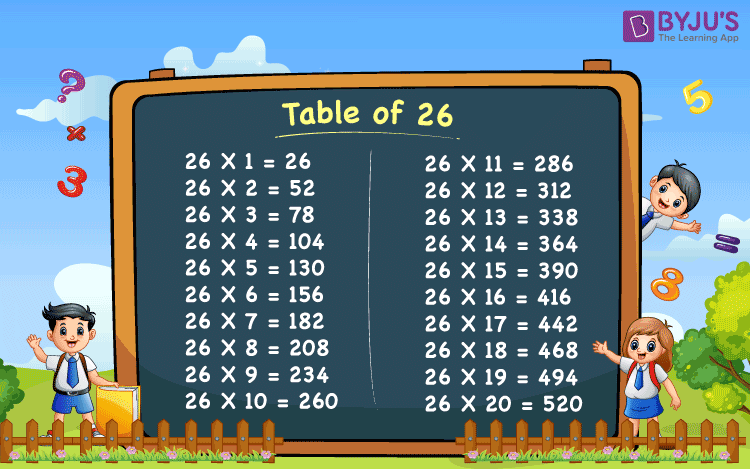# Table of 26

Tables of 26 is provided here up to 50 times. Tables are the basics of Mathematics based on which many multiplication calculations could be simplified quickly. Table of 26 gives the repeated addition of number 26, repeated for a certain number of times. For example, 4 baskets of 26 apples each, gives the sum of 26 + 26 + 26 + 26 = 104. Therefore, the total number of apples in all the 4 baskets are 104, or we can also write it as, 4 x 26 = 104, where 4 is the number of baskets and 26 is the number of apples in each basket.

## Table of 26 ChartThe multiplication tables are useful to all the level of students studying from Classes 1st to 12th.

By memorizing the tables 1 to 20, we can spare our time from consuming in typical multiplication problems. These tables can be used by candidates appearing for competitive examinations or common entrance test, which includes maths aptitude questions. The table of 26 is provided below which can be used for maths aptitude calculations.

## 26 Times Table

Let us draw the table of 26 from 1 to 50 times.

 26 x 1 = 26 26 x 2 = 52 26 x 3 = 78 26 x 4 = 104 26 x 5 = 130 26 x 6 = 156 26 x 7 = 182 26 x 8 = 208 26 x 9 = 234 26 x 10 = 260 26 x 11 = 286 26 x 12 = 312 26 x 13 = 338 26 x 14 = 364 26 x 15 = 390 26 x 16 = 416 26 x 17 = 442 26 x 18 = 468 26 x 19 = 494 26 x 20 = 520 26 x 21 = 546 26 x 22 = 572 26 x 23 = 598 26 x 24 = 624 26 x 25 = 650 26 x 26 = 676 26 x 27 = 702 26 x 28 = 728 26 x 29 = 754 26 x 30 = 780 26 x 31 = 806 26 x 32 = 832 26 x 33 = 858 26 x 34 = 884 26 x 35 = 910 26 x 36 = 936 26 x 37 = 962 26 x 38 = 988 26 x 39 = 1014 26 x 40 = 1040 26 x 41 = 1066 26 x 42 = 1092 26 x 43 = 1118 26 x 44 = 1144 26 x 45 = 1170 26 x 46 = 1196 26 x 47 = 1222 26 x 48 = 1248 26 x 49 = 1274 26 x 50 = 1300